Function Repository Resource:

# NonMaximumSuppression

Filter regions based on their overlap

Contributed by: Giulio Alessandrini
 ResourceFunction["NonMaximumSuppression"][{reg1,reg2,…}] keeps the rectangular regions regi with low overlap between each other. ResourceFunction["NonMaximumSuppression"][{reg1,…}→{w1,…}] uses the weights wi to score the importance of each regi. ResourceFunction["NonMaximumSuppression"][regions,property] gives the specified property for the filtered regions.

## Details and Options

ResourceFunction["NonMaximumSuppression"] is used to filter regions based on their mutual overlap and their weights.
Regions must be given in the form Rectangle[{xmin,ymin},{xmax,ymax}].
If the weights are not provided, regions are scored in linearly descending order with w1=1 and wn=1/n.
The following properties are supported:
 "Index" position of the selected region "Region" selected region "Weight" the weight used to score the region {prop1,…} a list of properties All all the available properties
The following options are supported:
 AcceptanceThreshold Automatic region acceptance threshold MaxOverlapFraction Automatic maximum allowed overlap fraction Method "Hard" method to use
Possible values for Method are:
 "Hard" remove regions with overlap greater than the specified threshold "Soft" iteratively rescore the regions based on their overlap

## Examples

### Basic Examples (2)

Filter out rectangles from list that have a big overlap with other rectangles in the list:

 In:=Out=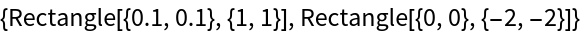Define and display a list of rectangle shapes:

 In:=Out=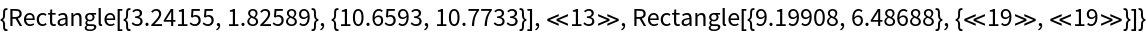In:=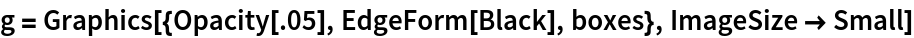Out=Delete rectangles with high overlap and display the results in red along with the original rectangles in black:

 In:=Out=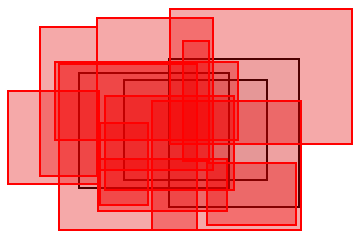Only keep non-overlapping rectangles:

 In:=Out=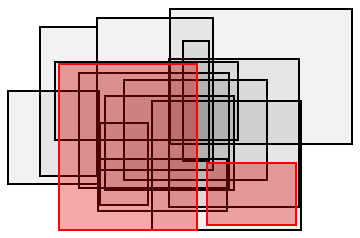### Scope (4)

Perform non-max suppression on a list of regions:

 In:=Out=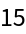In:=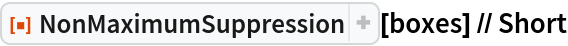Out=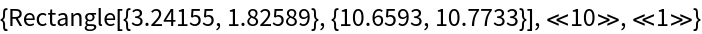In:=Out=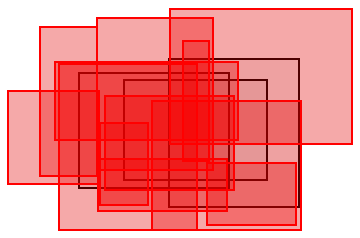Provide an explicit list of weights:

 In:=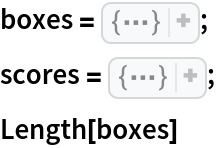Out=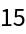Performing non-maximum suppression eliminated some of the rectangles:

 In:=Out=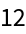Show the result:

 In:=Out=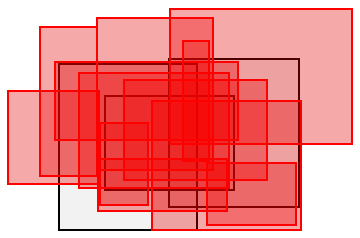In:=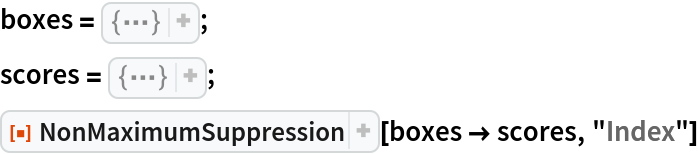Out=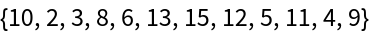The index is determined by the order of the scores for rectangles that contained in the result:

 In:=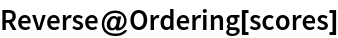Out=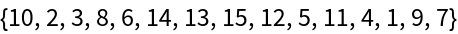Ask for a list of properties:

 In:=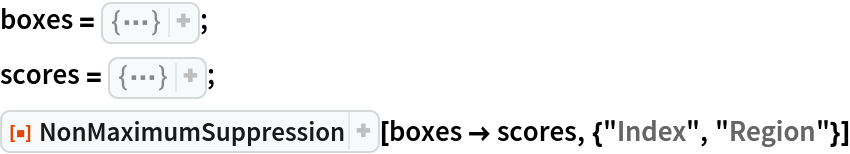Out=In:=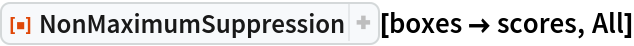Out=### Options (6)

#### AcceptanceThreshold (2)

Specify an AcceptanceThreshold for the regions:

 In:=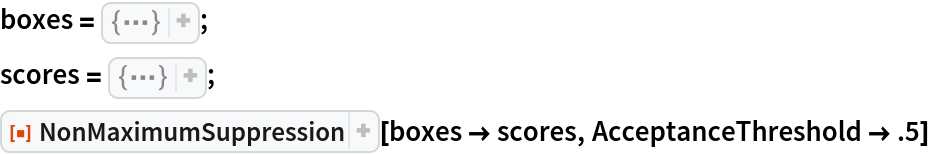Out=In:=Out=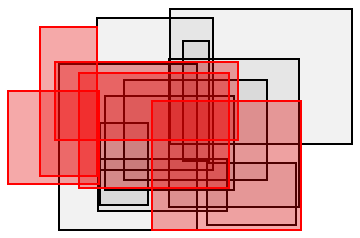Specify values to use before and after the overlap filtering:

 In:=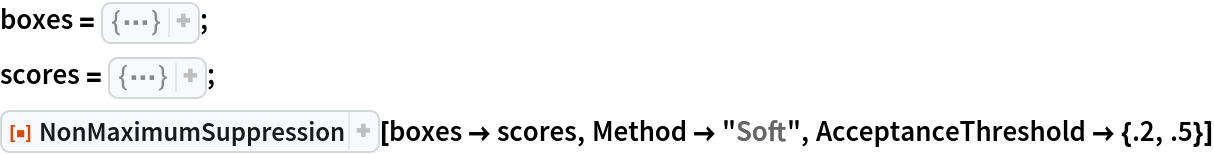Out=In:=Out=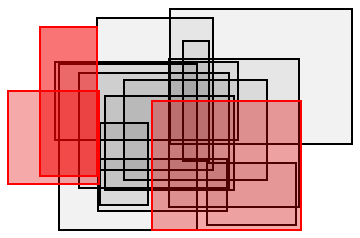#### MaxOverlapFraction (2)

Use to only keep regions with an overlap smaller than f:

 In:=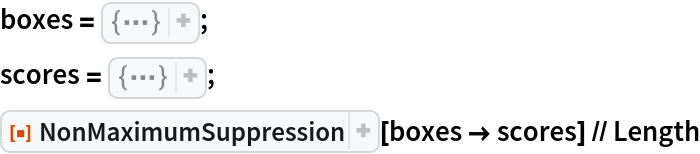Out=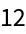In:=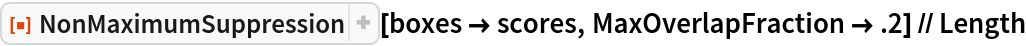Out=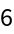If soft NMS is used, MaxOverlapFraction is used to defined the scaling behaviour:

 In:=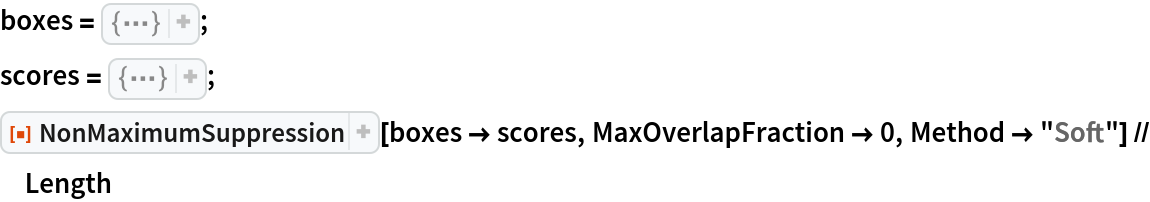Out=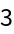If the a scaling value is given explicitly, the option is ignored:

 In:=Out=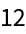#### Method (2)

By default, regions are removed using a hard overlap threshold:

 In:=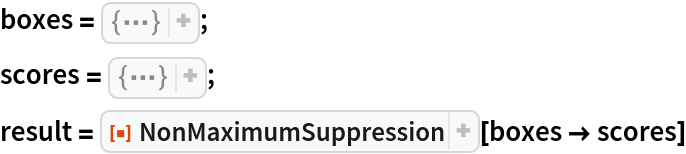Out=In:=Out=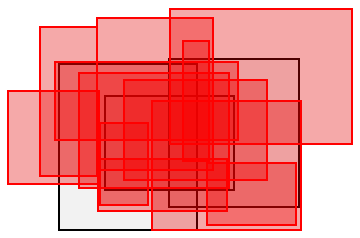Filter boxes using a soft NMS that scales the weights based on the overlap:

 In:=Out=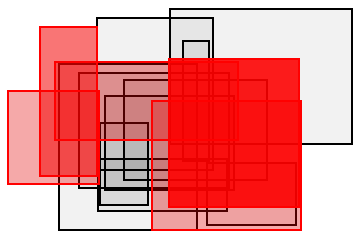Specify the scaling value used to update the regions weights:

 In:=Out=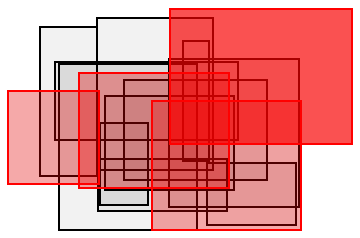Soft NMS can become very slow if boxes are not removed:

 In:=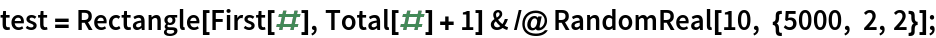In:=Out=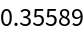In:=Out=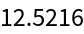Even a small threshold can significantly speed-up the computation:

 In:=Out=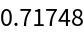## Version History

• 1.0.0 – 14 December 2021